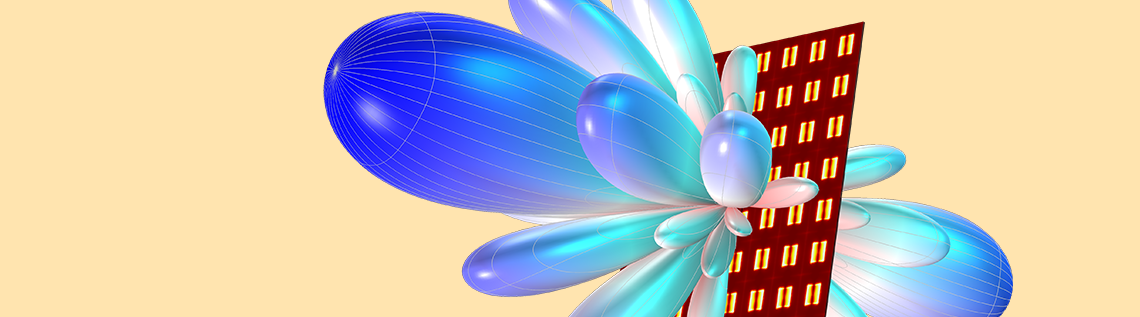# 如何合成天线阵列的辐射方向图

2019年 4月 4日

### 天线在物联网，IoS，SatCom 和 5G 中的应用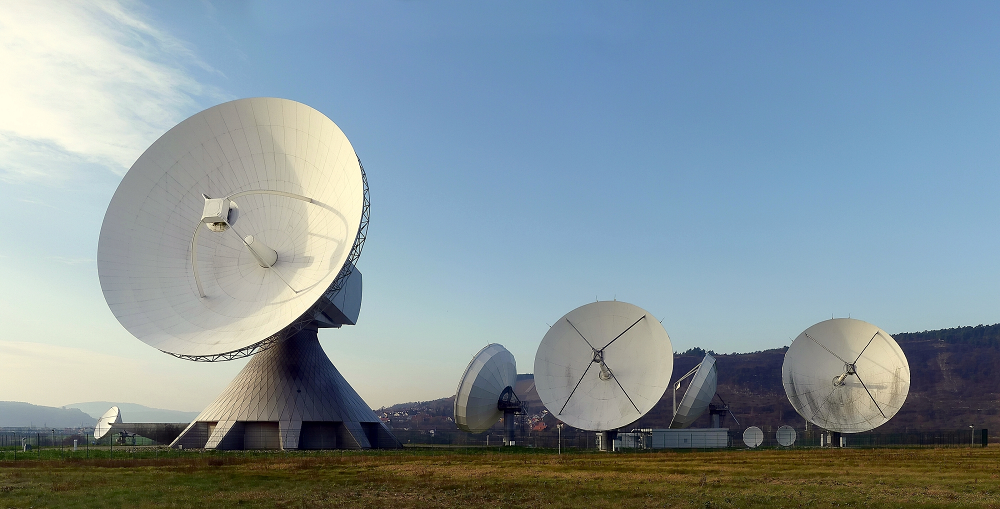### 使用阵列因子的好处

\frac{sin(\frac

{n_x (2 \pi d_x sin\theta cos\phi + \alpha_x)} {2})}{sin(\frac{2 \pi d_x sin\theta cos\phi + \alpha_x}{2}
)} \frac{sin(\frac

{n_y (2 \pi d_y sin\theta sin\phi + \alpha_y)} {2})}{sin(\frac{2 \pi d_y sin\theta sin\phi + \alpha_y}{2}
)} \frac{sin(\frac

{n_z (2 \pi d_z cos\theta + \alpha_z)} {2})}{sin(\frac{2 \pi d_z cos\theta + \alpha_z}{2}
)}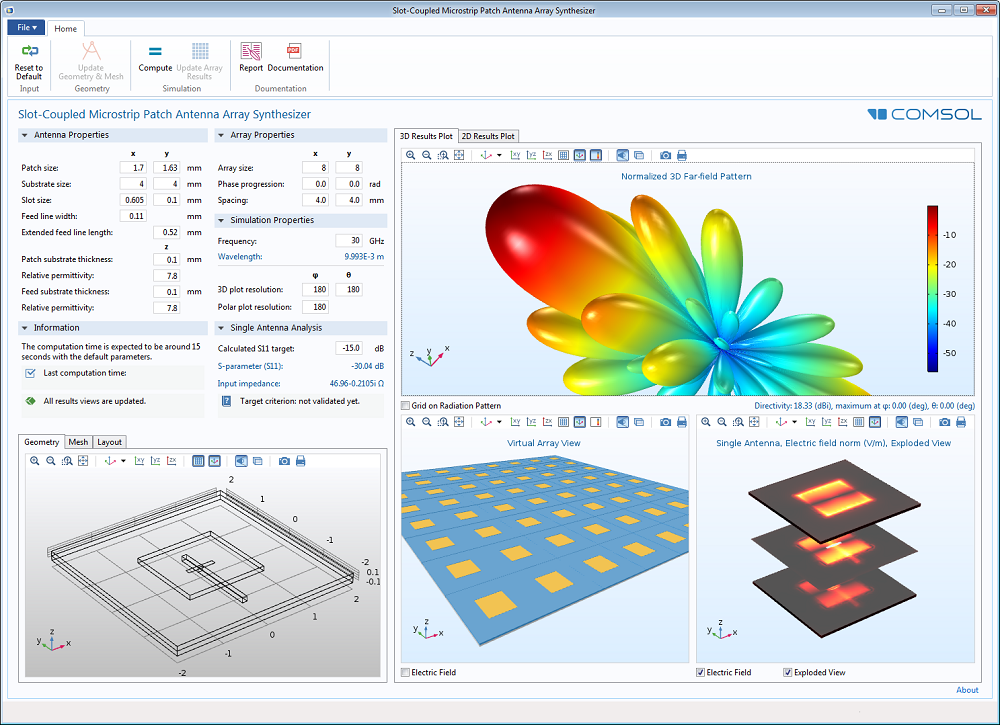### 射频模块中的阵列因子功能

af3(nx, ny, nz, dx, dy, dz, alphax, alphay, alphaz)

emw.gaindBEfar + 20 * log10（emw.af3（8，8，1，0.48，0.48，0，0，0，0））+ 10 * log10（1/64）

nx 沿 x 轴 的单元数 8.00 无量纲
ny 沿 y 轴 的单元数 8.00 无量纲
nz 沿 z 轴 的单元数 1.00 无量纲
dz 沿 x 轴的 阵列单元之间的距离 0.48 波长
dy 沿 y 轴的 阵列单元之间的距离 0.48 波长
dz 沿 z 轴的 阵列单元之间的距离 0 波长
alphax 沿 x 轴的 相传播 0 弧度
alphay 沿 y 轴的 相传播 0 弧度
alphaz 沿 z 轴的 相传播 0 弧度

\alpha_x=-kdcos\theta=\frac{2\pi d}

{\lambda}

cos\theta

-2 * pi * 0.48 * cos（pi / 3）

“ 案例库”中的  “微带贴片天线” 教程  展示了如何使用阵列因子来演化单个天线的辐射模式。

• 单个微带贴片天线的增益
• 模式设置为主瓣方向与 x 轴成 60 度和与 z 轴成30度的均匀阵列因子均匀的阵列因子
• 8×8 微带贴片天线阵列的合成增益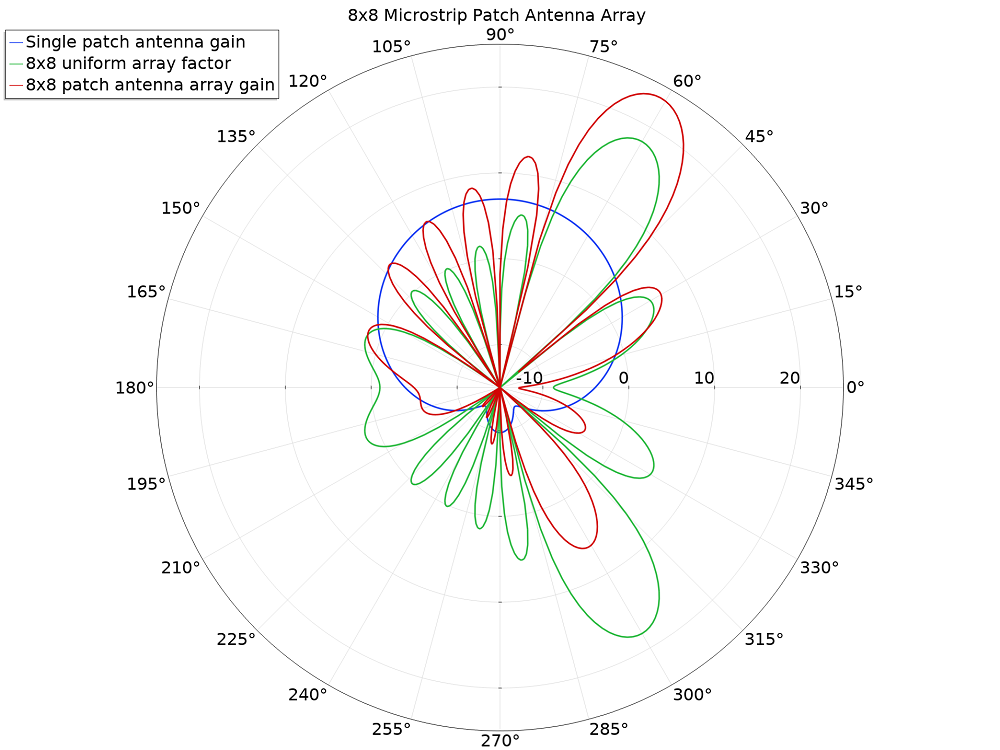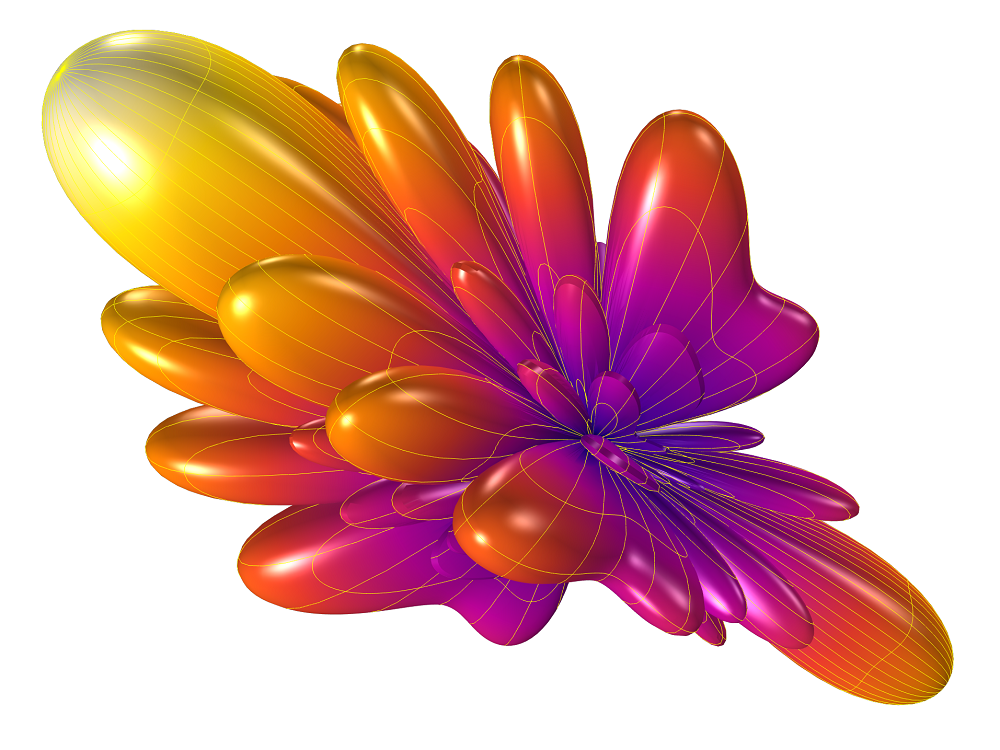### 天线阵列仿真的后处理

COMSOL Multiphysics中的各种后处理选项使您能够有效地研究天线原型。在动画设置中为动画序列类型使用 全谐波动态数据扩展 是一种非常有用的方法，可以在不仿真每个角度扫描点的情况下，检查波束转向的可行性，而无需在每个角度扫描点都运行模拟。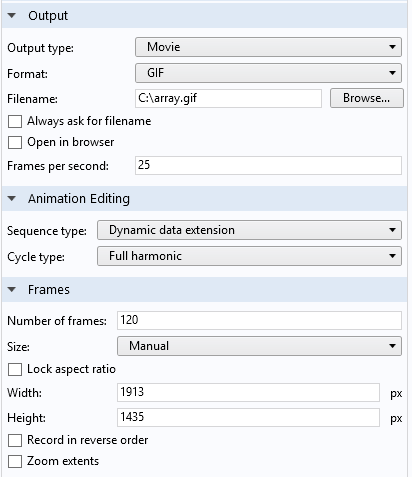l

emw.gaindBEfar + 20*log10(emw.af3(8, 8, 1, 0.48, 0.48, 0, -2*pi*0.48*cos(phase+pi/2), 0, 0)) + 10*log10(1/64)

8×8 微带贴片天线阵列的远场增益模式。主波束沿一个轴移动。

emw.gaindBEfar + 20*log10(emw.af3(12, 12, 1, 0.48, 0.48, 0, -2*pi*0.48*cos(pi/2-pi/8*cos(phase)), -2*pi*0.48*cos(pi/2-pi/8*sin(phase), 0)) + 10*log10(1/144)12×12 微带贴片天线阵列的远场增益模式。主波束沿圆形轨道移动。